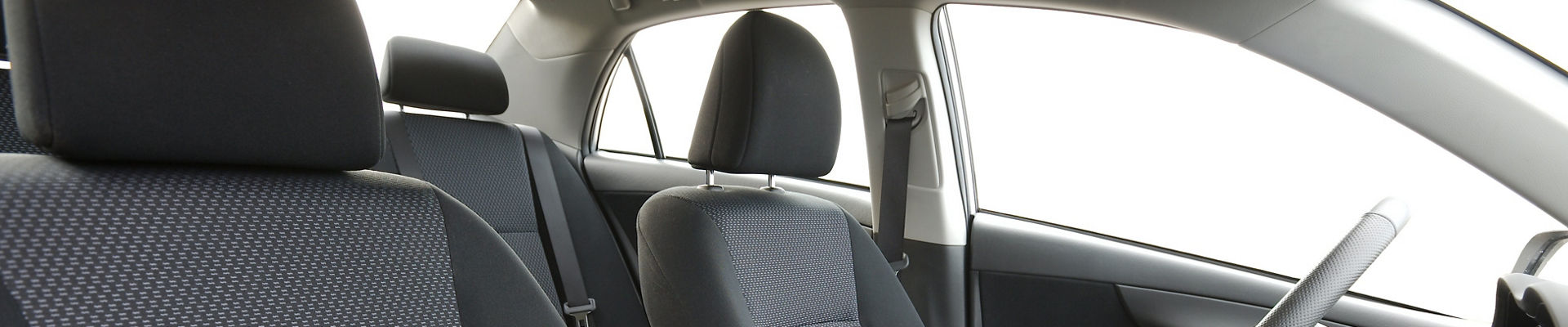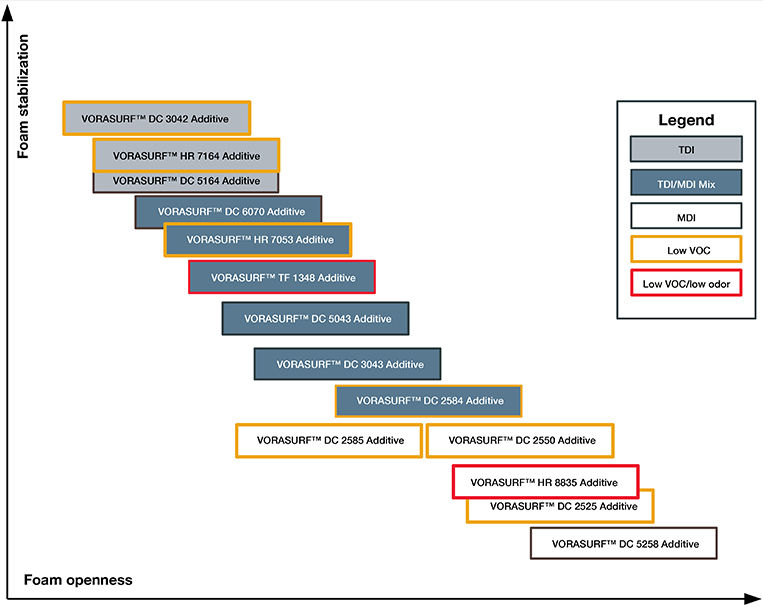• Montenegro
• St.Pier,Miquel.
• Vietnam
• 不丹
• 东帝汶岛
• 中非共和国
• 丹麦
• 乌克兰
• 乌兹别克斯坦
• 乌干达
• 乌拉圭
• 乍得
• 乔治亚
• 也门
• 亚美尼亚
• 以色列
• 伊拉克
• 伯利兹
• 佛得角群岛
• 俄罗斯联邦
• 保加利亚
• 克罗地亚
• 关岛
• 冈比亚
• 冰岛
• 几内亚
• 几内亚比绍
• 列支敦士登
• 刚果共和国
• 刚果民主共和国
• 利比里亚
• 加拿大
• 加纳
• 加蓬
• 匈牙利
• 北马其顿
• 北马里亚纳群岛
• 南三维治岛
• 南极洲
• 南非
• 博内尔、萨巴群岛
• 博茨瓦纳
• 卡塔尔
• 卢旺达
• 卢森堡
• 印度
• 印度尼西亚
• 危地马拉
• 厄瓜多尔
• 吉尔吉斯斯坦
• 吉布提
• 哈萨克斯坦
• 哥伦比亚
• 哥斯答黎加
• 喀麦隆
• 图瓦卢
• 土库曼斯坦
• 土耳其
• 圣基茨和那维斯
• 圣多马 普林西比
• 圣文森特
• 圣诞岛
• 圣赫勒拿岛
• 圣路西亚
• 圣马丁
• 圣马力诺
• 圭亚那
• 坦桑尼亚
• 埃及
• 埃塞俄比亚
• 埃立特里亚
• 基里巴斯
• 塔吉克斯坦
• 塞内加尔
• 塞尔维亚
• 塞拉利昂
• 塞浦路斯
• 塞舌尔群岛
• 墨西哥
• 多哥
• 多米尼加
• 多米尼加共和国
• 大中华区 (中国大陆)
• 大中华区 (台湾)
• 大中华区 (澳门特别行政区)
• 大中华区 (香港特别行政区)
• 奥地利
• 委内瑞拉
• 孟加拉国
• 安哥拉
• 安圭拉岛
• 安提瓜和巴布达
• 安道尔共和国
• 密克罗尼西亚
• 小奥特兰群岛
• 尼加拉瓜
• 尼日利亚
• 尼日尔
• 尼泊尔
• 巴哈马(群岛)
• 巴基斯坦
• 巴巴多斯
• 巴布亚新几内亚
• 巴拉圭
• 巴拿马
• 巴林
• 巴西
• 布基纳法索
• 布维群岛
• 布隆迪
• 希腊
• 帕劳
• 库拉索
• 库科纳群岛
• 开曼群岛
• 德国
• 意大利
• 所罗门群岛
• 托客劳群岛
• 拉脱维亚
• 挪威
• 捷克共和国
• 摩尔多瓦
• 摩洛哥
• 摩纳哥
• 撒哈拉西部
• 文莱达鲁萨兰
• 斐济
• 斯威士兰
• 斯洛伐克
• 斯洛文尼亚
• 斯里兰卡
• 新加坡
• 新喀里多尼亚
• 新西兰
• 日本
• 智利
• 柬埔寨
• 格林纳达
• 格陵兰岛
• 梵蒂冈城
• 比利时
• 毛里塔尼亚
• 毛里求斯
• 汤加
• 沙特阿拉伯
• 法国
• 法国南部地区
• 法属圭亚那
• 法属波利尼西亚
• 法罗群岛
• 波兰
• 波多黎哥
• 波黑
• 泰国
• 津巴布韦
• 洪都拉斯
• 海地
• 澳大利亚
• 爱尔兰
• 爱沙尼亚
• 牙买加
• 特克斯凯科斯
• 特立尼达 多巴哥
• 玻利维亚
• 瑙鲁
• 瑞典
• 瑞士
• 瓜达洛普
• 瓦利斯 富图纳岛
• 瓦努阿图
• 白俄罗斯
• 百慕大群岛
• 皮特肯岛
• 皮特肯岛
• 直布罗陀
• 福克兰群岛
• 科克群岛
• 科威特
• 科摩罗群岛
• 科索沃
• 秘鲁
• 突尼斯
• 立陶宛
• 索马里
• 约旦
• 纳米比亚
• 纽埃群岛
• 统一
• 缅甸
• 罗马尼亚
• 美国
• 美属维尔京群岛
• 老挝
• 肯尼亚
• 芬兰
• 苏里南
• 英国
• 英属印度洋区
• 英属维尔京群岛
• 荷 兰
• 荷德 马克多纳岛
• 莫桑比克
• 莱索托
• 菲律宾
• 萨尔瓦多
• 萨摩亚
• 萨摩亚, 美国
• 葡萄牙
• 蒙古
• 蒙塞拉特岛
• 西班牙
• 诺福克岛
• 象牙海岸
• 贝宁
• 赞比亚
• 赤道几内亚
• 阿塞拜疆
• 阿富汗
• 阿尔及利亚
• 阿尔巴尼亚
• 阿曼
• 阿根廷
• 阿联酋
• 阿鲁巴
• 韩国
• 马尔代夫
• 马拉维
• 马提尼克
• 马来西亚
• 马约特岛
• 马绍尔群岛
• 马耳他
• 马达加斯加
• 马里
• 黎巴嫩# 模塑聚氨酯泡沫添加剂## 模塑泡沫添加剂，可提高质量、性能和创新性

• MDI和TDI模塑泡沫系统
• 冷固化和高密度MDI模塑泡沫系
• 吸能NVH系统
 PRODUCT MDI TDI/MDI TDI 低排放 推荐助表面活性剂 效力 推荐  使用 (PPH） VORASURF™ DC 3042 添加剂 - - ✓✓✓ +++ 是的。 极高 0.2-0.6 VORASURF™ DC 5164 添加剂 - - ✓✓✓ - 是的。 非常高 0.2-1.0 VORASURF™ DC 6070 添加剂 - ✓✓✓ ✓✓✓ - - 中高 0.5-0.8 VORASURF™ HR 7053 添加剂 - ✓✓✓ ✓✓✓ +++ - 中高 0.2-0.8 VORASURF™ TF 1348 添加剂 - ✓✓✓ ✓✓✓ +++ - 中高 0.2-0.8 VORASURF™ DC 5043 添加剂 - ✓✓✓ ✓✓ - - 中等 0.5-1.0 VORASURF™ DC 3043 添加剂 - ✓✓ ••• - - 高 0.5-1.0 VORASURF™ DC 2584 添加剂 ✓✓ ✓✓ •• + - 中高 0.5-1.0 VORASURF™ DC 2585 添加剂 ✓✓✓ - - ++ - 中等 0.5-1.0

••• 强烈建议用作助表面活性剂 •• 建议用作助表面活性剂 • 可用作助表面活性剂

？强烈推荐用于应用？推荐用于应用？ 可用于应用